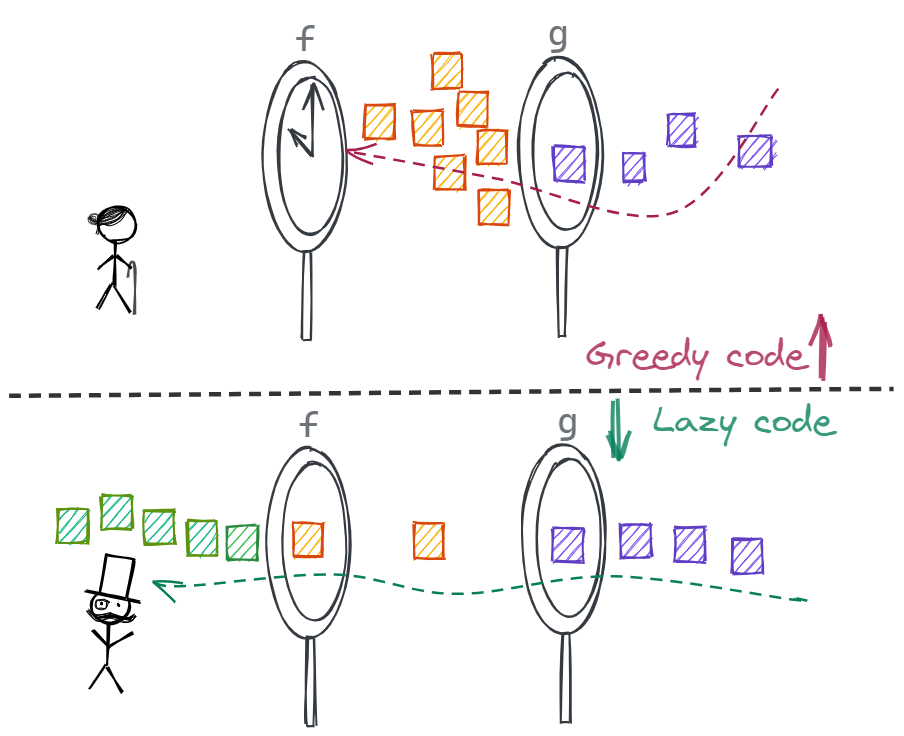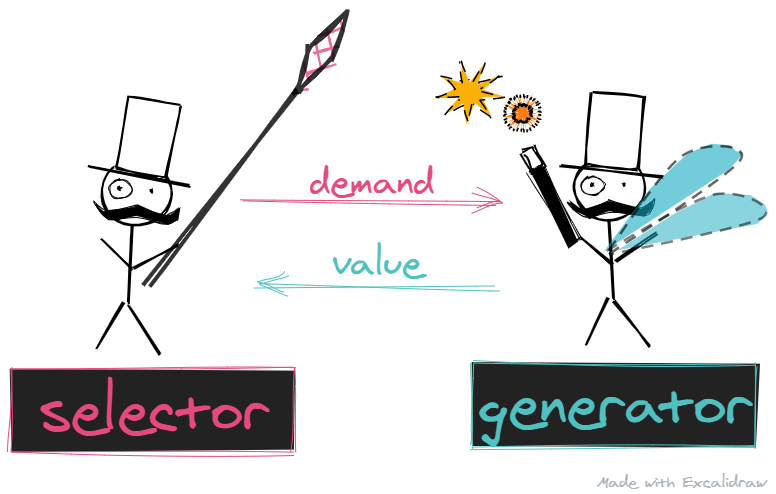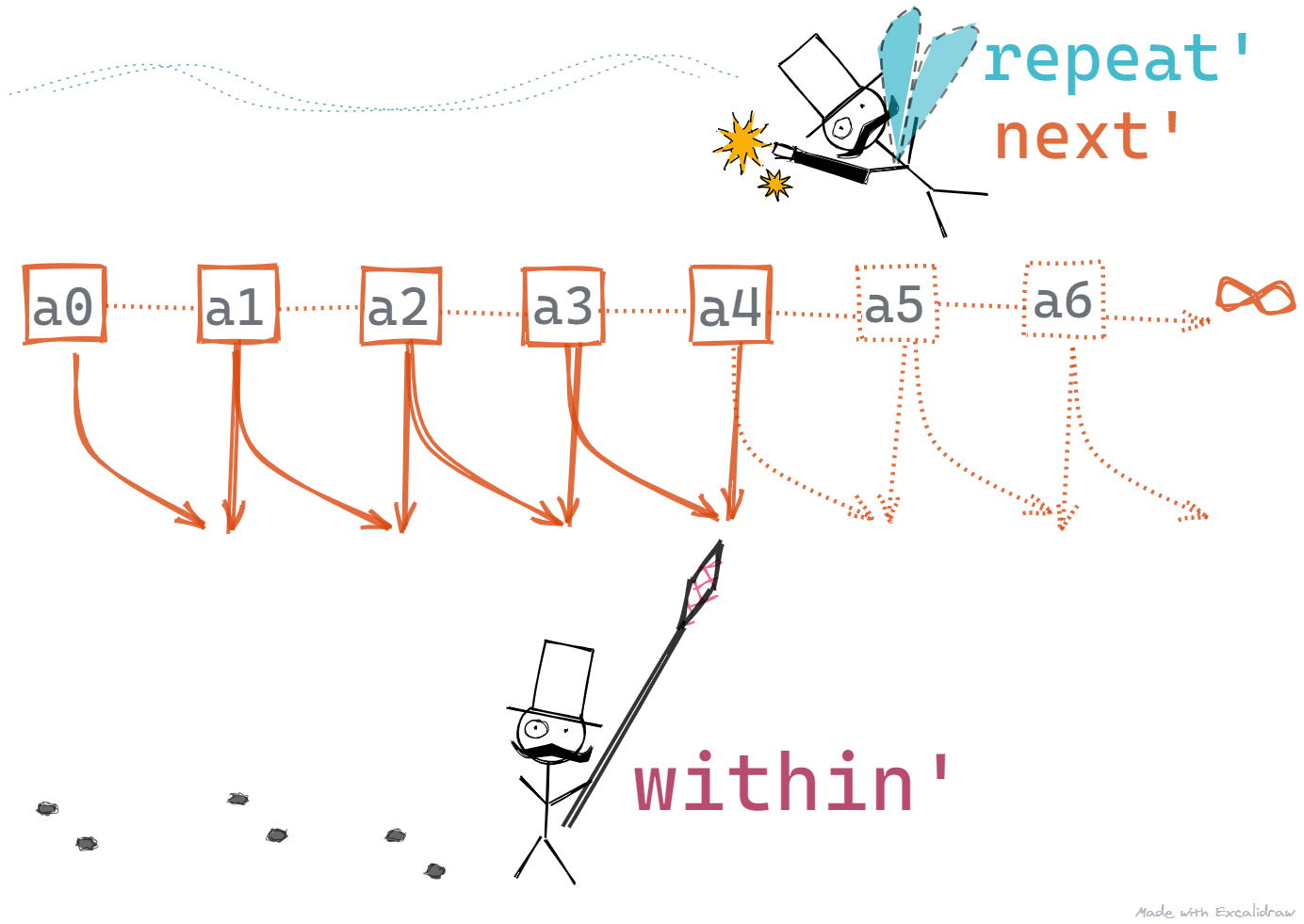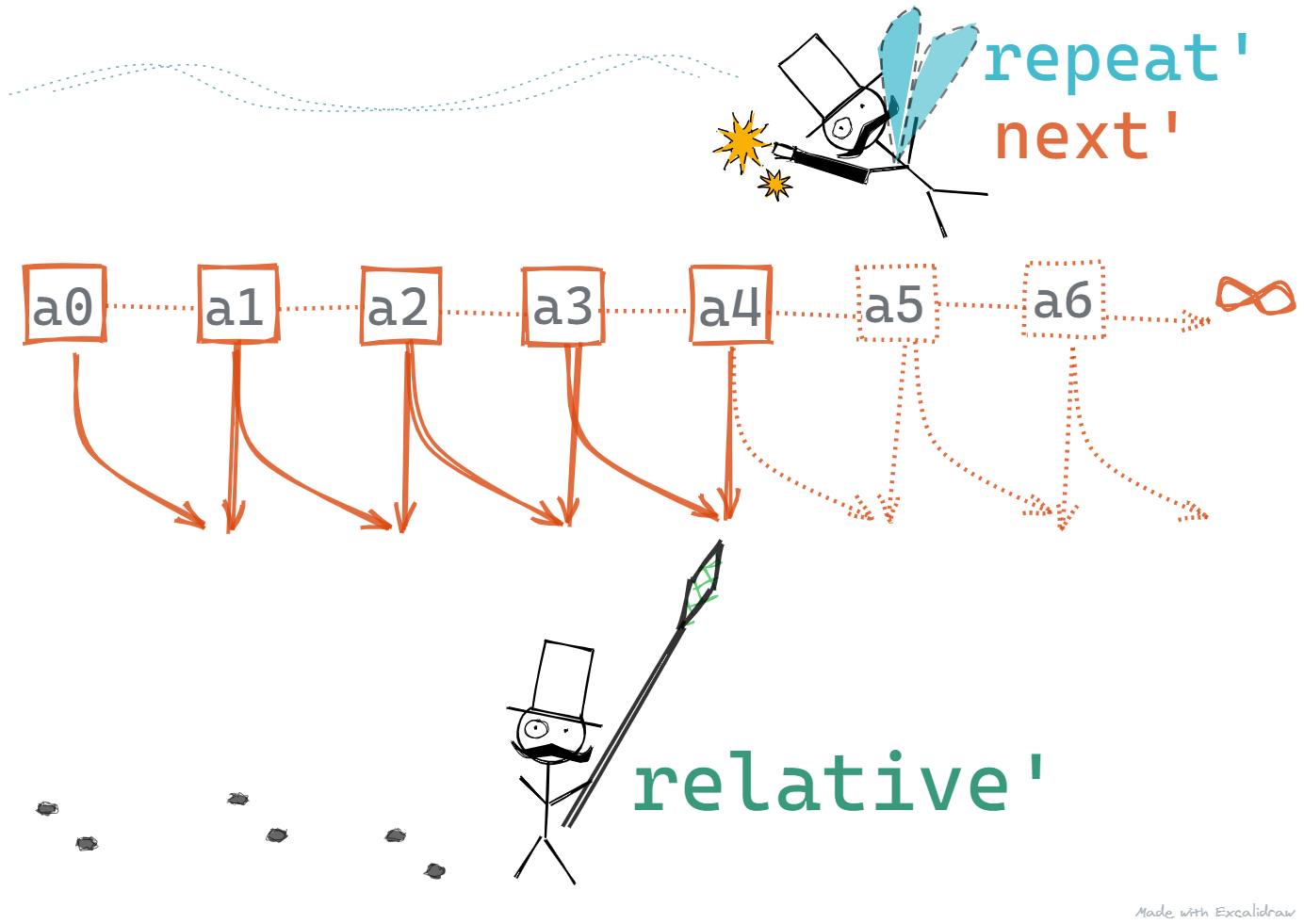# Why FP Matters - Part 3 🎩Picture by freddie marriage

Lazy evaluation - Conjuring infinities

# A Quick Recap

We have been following the white paper by John Hughes1. And so far we've touched upon the below.

1. Why FP Matters - Part 1 🐘 : A brief introduction - How FP can help improve developer productivity and the motivation for popular languages to adapt functional aspects.
2. Why FP Matters - Part 1½ 💫: A bit of Haskell - A bit of Haskell to get used to the syntax and the syntactic sugar.
3. Why FP Matters - Part 2 🎈: Higher-order functions - Basics of higher-order functions, partial application and currying.
4. Why FP Matters - Part 2½ 🍰 - Slicing complexity with Higher-order functions - How to develop powerful and reusable functions to process lists and trees to demonstrate the power of higher-order functions.

# What we will cover in this post?

We've been talking about how functional programming is different because it lets us break down complexity in different ways. We mentioned that this is possible because of two main types of glues i.e. the way we put things together after breaking them down. We were introduced to the first glue - higher-order functions in the last two posts. It helped us build re-usable functions.

In this one, we will cover lazy evaluation to see how we can put functions together and how it is different from the traditional way to program.

We will write programs that use Numerical Methods to calculate the square root of numbers. There will be code!

# Composing ProgramsPicture by Katherine Hanlon

We've already looked at the composition operator briefly in the previous post. Let's do a little refresher. The `.` (dot) or the compose operator can be used to glue functions together.

`(f . g) x`

will act as

`f (g x)`

Simply put, if we pass input to the expression `(f . g)`, the input will be first passed to the function `g` which will return an output that will be the input to the function `f`.

Prima facie, this looks like something we can do with traditional imperative programs. It is a little different though. Imperative programs are greedy, when `g` is applied to the input. It has to finish processing the input completely before it can hand over the execution to the function `f`. This leads to the below

• `g` might, and in most cases will save the output temporarily in memory or on disk storage if it gets too large. If we have several of these programs glued together, it will mean multiple temporary storages.
• If the temporary storage required gets too large, the programs cannot be glued together.
• Processing of infinite sequences becomes difficult and almost impossible if we are limited this way. You might say that we can still put together the behavior for two functions to process our never-ending stream, but we hardly deal any solution that has just two functions, and programs need to be dynamic and easy to change.
• The net effect of this leads to cod rot since a single module or a function accumulates multiple responsibilities over time.

On the other hand, with functional programs, we have can use lazy evaluation to avoid bottlenecks and let the data flow through, as it is being processed.It sounds counter-intuitive, doesn't it? Let's have a look to understand how this works.

Functional languages provide a solution to this problem by being lazy and running the program together in strict synchronization.

In the expression `f . g`, the execution of `g` and `f` are interdependent

• `g` starts running only when `f` tries to read an input, `g` stops running as soon as `f` stops. In other words, `g` runs only for `f`. `g` is lazy
• When `f` starts, it is suspended until `g` tries to read and input. This is what we mean by strict synchronization.
• If due to some condition, `f` decides to terminate before reading all the inputs from `g`, then `g` terminates too. This allows `g` to be non-terminating. It can produce an infinite amount of outputs and we can rely on `f` to terminate it as soon as it is done.This allows for programs to be modularized differently. Loops can be thought of as made up of two parts - Selectors and Generators

• Termination conditions OR Selectors - the gentleman with the net
• Selectors are responsible for selecting values
• They can demand values from Generators
• Once they've demanded, they wait patiently until they receive a value
• Loop bodies OR Generators - the fairy
• Generators are responsible for generating values
• They only generate values on-demand
• Generators do not generate values on their own
• Generators, may produce an infinite number of values

This ends up being very powerful, as we will see in a bit.

# 🥕 Finding RootsPicture by Nguyen Dang Hoang Nhu

It is simple to find a square of a number, but if we want to find a square root from a square, there is no formula for it. We have to rely on approximations, and we know of some methods to better the approximations iteratively, until we are satisfied with the accuracy of our approximation.

One such method is the Newton-Raphson Method.

A Short Proof

The Newton-Raphson method for finding the square root of a number states that for a number `N`, we can start with an approximation `a_n`, and find a better approximation `a_n+1` using the below equation.

`a_n+1 = (a_n + N / a_n) / 2`

So,

• `a_1 = (a_0 + N / a_0) / 2`
• `a_2 = (a_1 + N / a_1) / 2`
• `a_3 = (a_2 + N / a_2) / 2`, and so on.

At some point, we will have `a_n` almost equal to `a_n+1`, and using this we can simply rewrite `a_n+1 = (a_n + N / a_n) / 2` as

`a = (a + N / a) / 2`

• `2*a = a + N / a`, multiplying both sides by `2`
• `a = N / a`, substracting both sides by `a`
• `a*a = N`, multiplying both sides by `a`
• `a = √N`, square root both sides

That checks out! Now let's look at some code that is written imperatively to use the above algorithm.

⚠ Imperative Code

`// JavaScriptvar sqrt = function (n, a0, eps) {  var previous = a0 + 2 * eps;  var current = a0;  do {    previous = current;    current = (current + n / current) / 2;    if (Math.abs(current - previous) < eps) {      break;    }  } while (true);  return current;};// sqrt(2)`

This code runs fine on its own but it combines several responsibilities

• `current = (current + n / current) / 2` - obtaining the next approximation from the current
• `if (Math.abs(current - previous) < eps) { ... }` - the termination condition
• `do { ... }` - the looping body

If any of the above changes, our function will change. The function is also not extensible.

NOTE: I am using JavaScript, but this could be any other language. I chose JavaScript because it is less noisy and should be easier to read for most folks. Also, please note that JavaScript doesn't make it compulsory to write code this way.

Let's get back to Haskell and try a more decoupled and more modular approach. We will define three separate methods

`next'` - A simple function that has one responsibility. Take in the number whose square root we want to find along with an approximation of the square root value and simply return the next approximation using the Newton-Raphson method.

`next' n x = (x + n / x) / 2`

`repeat'` - It takes in a function `f` along with a value `a`, and it simply returns `a` prepended to the result obtained by calling itself again with the same function `f`. Only this time, we pass in `f a`. i.e. a transformed value of `a`. It is a recursive definition.

Basically, this series - `[a0 : f a0 : f(f a0) : f(f(f a0)) : …]`

`repeat' f a = a : repeat' f (f a)`

Note: In the above definition, each time repeat is called

• only a part of the expression is evaluated. The value of `a` is prepended to a list which is just an expression `repeat' f (f a)` - lazy
• the second parameter to `repeat'` i.e. `f a` is evaluated - greedy
`double x = x + xrepeat' double 1`

When we execute the above code, It will return, `1 : 2 : 4 : 8 : 16 : 32 ... ` and so on until `infinity`. This is because it assumes that we want the values.

Yes, it will crash your program, and yes, it looks dangerous. But we won't let it run on like that. And by the way, if you hadn't noticed. This is our Generator.

`within'` - This function, takes in an acceptable error margin `eps` and a list of values. Then it simply,

• takes in the first two values of the input sequence and checks if they are within the acceptable range of error
• if this condition is satisfied, then the second value is returned as a result
• otherwise, it drops the first value from the list and calls itself with the rest of the input sequence Again, a recursive definition.
`within' eps (a1:a2:rest)  | (abs (a1 – a2)) < eps = a2  | otherwise = within' eps (a2:rest)`

Note: `within'` uses a Haskell feature called guards, it is just a cleaner, and more math-like way to write conditional logic.

When we compose `repeat'` with `within'`,

• `repeat'` only returns a value when `within'` demands one from it, and
• when `within'` is satisfied and terminates, `repeat'` terminates too.

`within'` acts as our Selector.

So when our program begins, we don't have any values present.

• Our selector - `within'` - moves through the list, starting at the beginning, demanding a couple of values at a time.
• And as it demands, our generator - `repeat' next'` - keeps generating values.Below are all the pieces of this puzzle put together for easy viewing.

`next' n x = (x + n / x) / 2repeat' f a = a : repeat' f (f a)within' eps (a1:a2:rest)  | (abs (a1 – a2)) < eps = a2  | otherwise = within' eps (a2:rest)sqrt' n a0 eps =  within' eps (repeat' (next' n) a0)sqrt' 2 1 10e-7 --1.414213562373095`

Twist in the tale

Our code works and it provides accurate results, mostly. We see a problem. When we are close enough to our result, the acceptable error margin i.e. the difference between two subsequent approximations is 10e-7. What if our number itself is smaller than that? We may get results that may be relatively further away than we need.

What do we do? Simple. We write a different selector.

`relative' eps (a1:a2:rest)  | (abs (a1 – a2)) < eps*(abs a2) = a2  | otherwise = relative' eps a2:restrelativeSqrt' n a0 eps =  relative' eps \$ repeat' (next' n) a0`

`relative'` - this function, takes in an acceptable error margin `eps` and a list of values and is similar to `within'`, except for the fact that it checks for relative difference and not just the difference in values.# 📝 Conclusion

"While some other systems allow programs to be run together in this manner, only functional languages (and not even all of them) use lazy evaluation uniformly for every function call"

-- Why Functional Programming Matters

This is true and it limits what you can do with lazy evaluation in the languages that don't full support lazy. Haskell is fully lazy but we can implement lazy behavior in JavaScript and other languages by relying on higher-order functions and closures. Under the hood, it is all functions.

"... lazy evaluation’s power depends on the programmer giving up any direct control over the order in which the parts of a program are executed, it would make programming with side effects rather difficult, because predicting in what order —or even whether— they might take place would require knowing a lot about the context in which they are embedded."

-- Why Functional Programming Matters

If you look back at our imperative example, it changed the values of variables. Other imperative programs may end up depending on the global state, this makes composing programs difficult and more difficult since functions will get coupled due to their dependency or impact on the environment outside their scope.

# Summary

1. We talked about composing programs in functional languages is different from the traditional way.
2. We were introduced to lazy evaluation, and how coupled with strict synchronization, it helps us deal with infinite sequences.
3. We learned about selectors and generators
4. We wrote code to find the square root of numbers in a modular fashion using all of the above.

Yet again, we saw how using functional programming helps us write re-usable components and how we can do a lot more with a lot less code. Next week we will see more power as we tame intertwined infinite sequences!

If you are interested in dabbling around with Haskell, you have the below options.

If you don't want to get your hands dirty, that's fine too. We'll continue here with the next one in this series - Why FP Matters.

# Up Next

Why FP Matters - Part 3½ 🧶: Weaving infinities We will see how lazy evaluation helps us to handle code with yet more increasing complexity.

Until then, have a happy new year, and see you on the other side! ⭐🌟✨

For more fun stuff like this, click here to see a list of all my posts1. Why Functional Programming Matters - http://www.cse.chalmers.se/~rjmh/Papers/whyfp.html